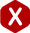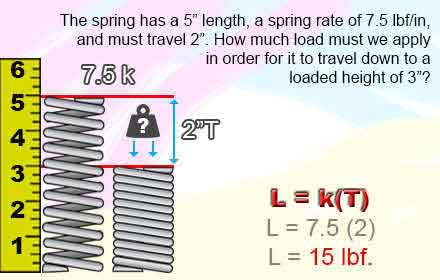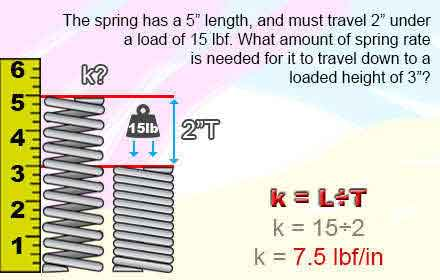<# Spring Load and Spring Rate

### Spring Rate Definition

#### The constant amount of force you need to travel ONE inch or millimeter of distance.

As it was explained in the definitions above, spring load determines a specific amount force or pressure at a specific loaded height while spring rate determines the rate of force you will need in order to travel one unit of measurement (in. or mm.). You can use either one to calculate the other; rate to calculate working loads and working loads to calculate rate. The formulas and diagrams are explained in simple examples below.

This example shows you how spring load works and how it is derived from spring rate. You have a spring with a free length of 5 inches and your spring rate is of 7.5 pounds of force per inch (lbf/in). This spring needs to reach a solid height of 3 inches, therefore having to travel 2 inches. To calculate the working load of how much force you’ll need in order to reach a solid height of 3 inches, you’ll have to multiply the distance traveled by the spring rate as shown in the following formula and diagram.

k(T)=L

7.5(2)=L
15 = L

### Example### Spring Rate

Spring rate is a calculation more complex to calculate; you’ll find the full formula at the bottom of this article. You can calculate your required spring rate based on your spring’s working loads too though. Take the values from the previous example. This way, you may also confirm the results you received on the previous formula. The distance traveled was of 2 inches while the load was 15 pounds of force. To calculate the required spring rate to meet these working loads you’ll have to divide the load by the distance traveled as shown in the following diagram and formula.

k= L ÷ T

k = 15 ÷ 2
k = 7.5 lb/in

### Example#### Full Spring Rate Formula

k = Gd^4 / [8^3D na]
G = E / 2(1 + V)
d = D outer - d

#### Formula Variables

• Spring Wire Diameter = d
• Spring Outside of Spring = D outer
• Mean Diameter Of Spring = D
• Young's Modulus of material = E
• shear modulus of material = G
• Spring Constant = K
• Active Coils = na
• Poisson ratio off Material = V